# NCERT Exemplar Solutions for Class 7 Maths Chapter 3 Data Handling

NCERT Exemplar Solutions for Class 7 Maths Chapter 3 Data Handling are available here. Maths is a subject which requires understanding and applying reasoning skills with logic. Along with this, it also requires students to practice Maths regularly. Students of Class 7 are suggested to solve NCERT Exemplar Solutions for Class 7 Maths.

Chapter 3 – Data Handling Solutions are available on this page. In various fields, we need information in the form of numerical figures. Each figure of this kind is called an observation. The collection of all the observations is called data. Now, let us have a look at the important topics discussed in the NCERT Exemplar Solutions for Class 7 Maths Chapter 3.

• Collection of Data
• Organisation of Data
• Arithmetic Mean
• Mode
• Mode of Large Data
• Median
• Bar Graphs
• Probability

## Download the PDF of NCERT Exemplar Solutions for Class 7 Maths Chapter 3 Data Handling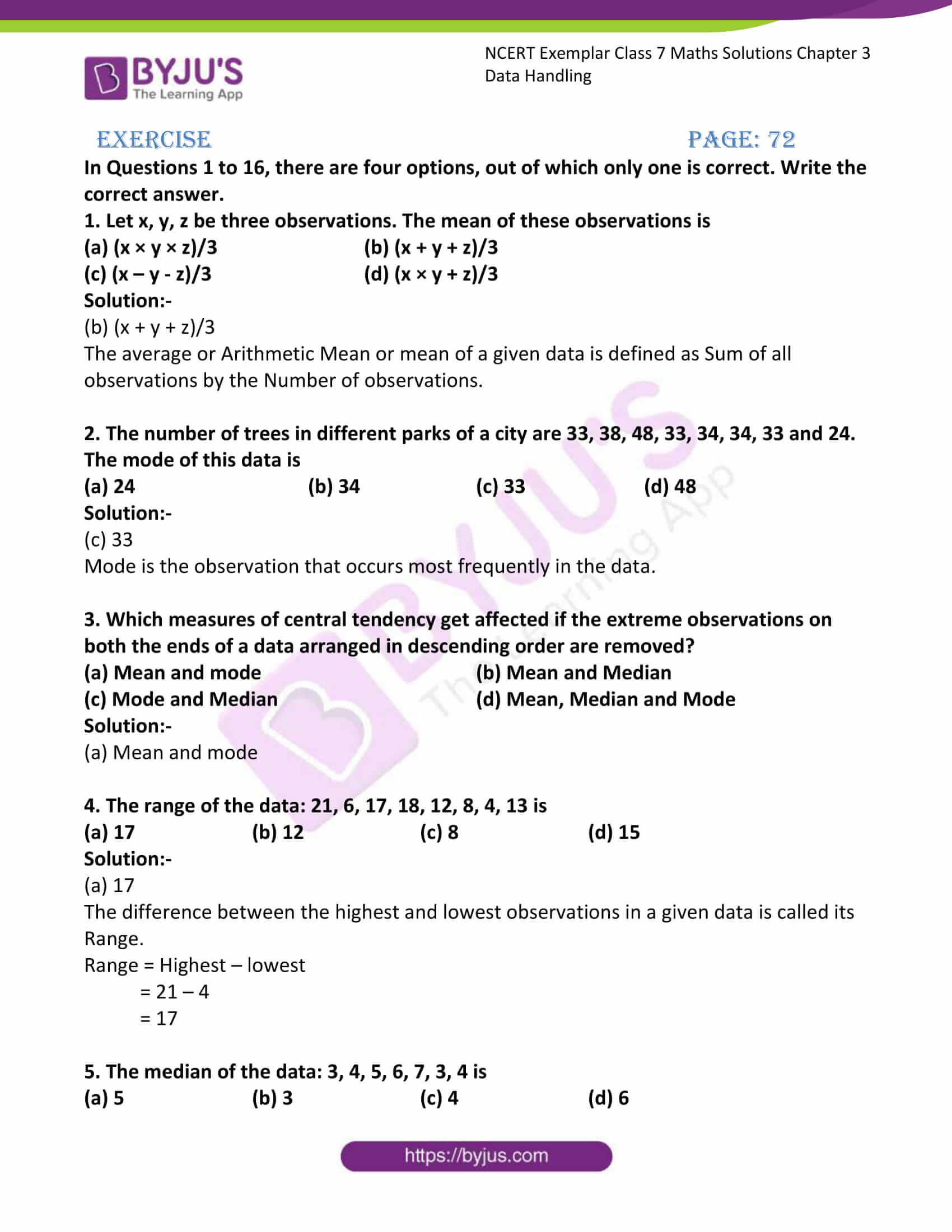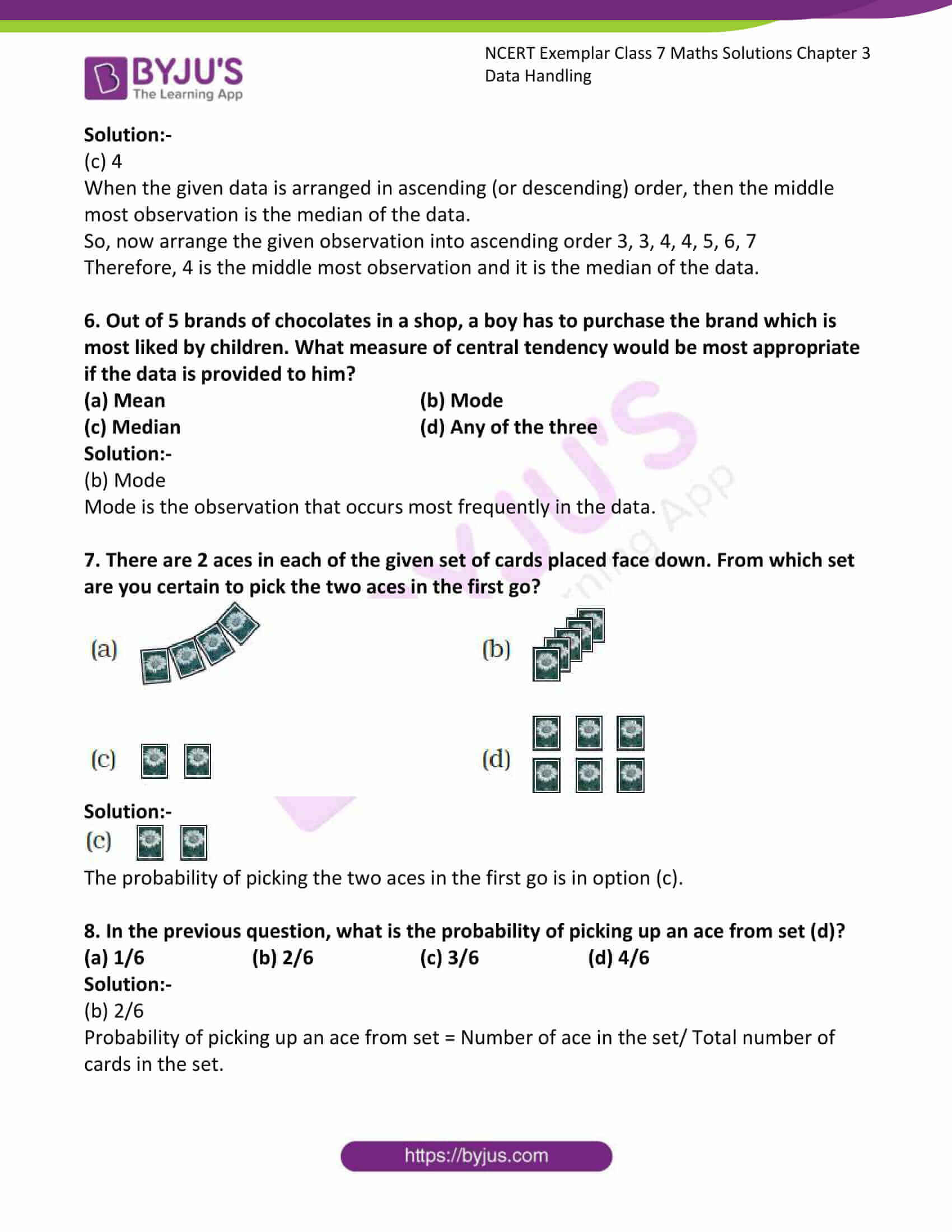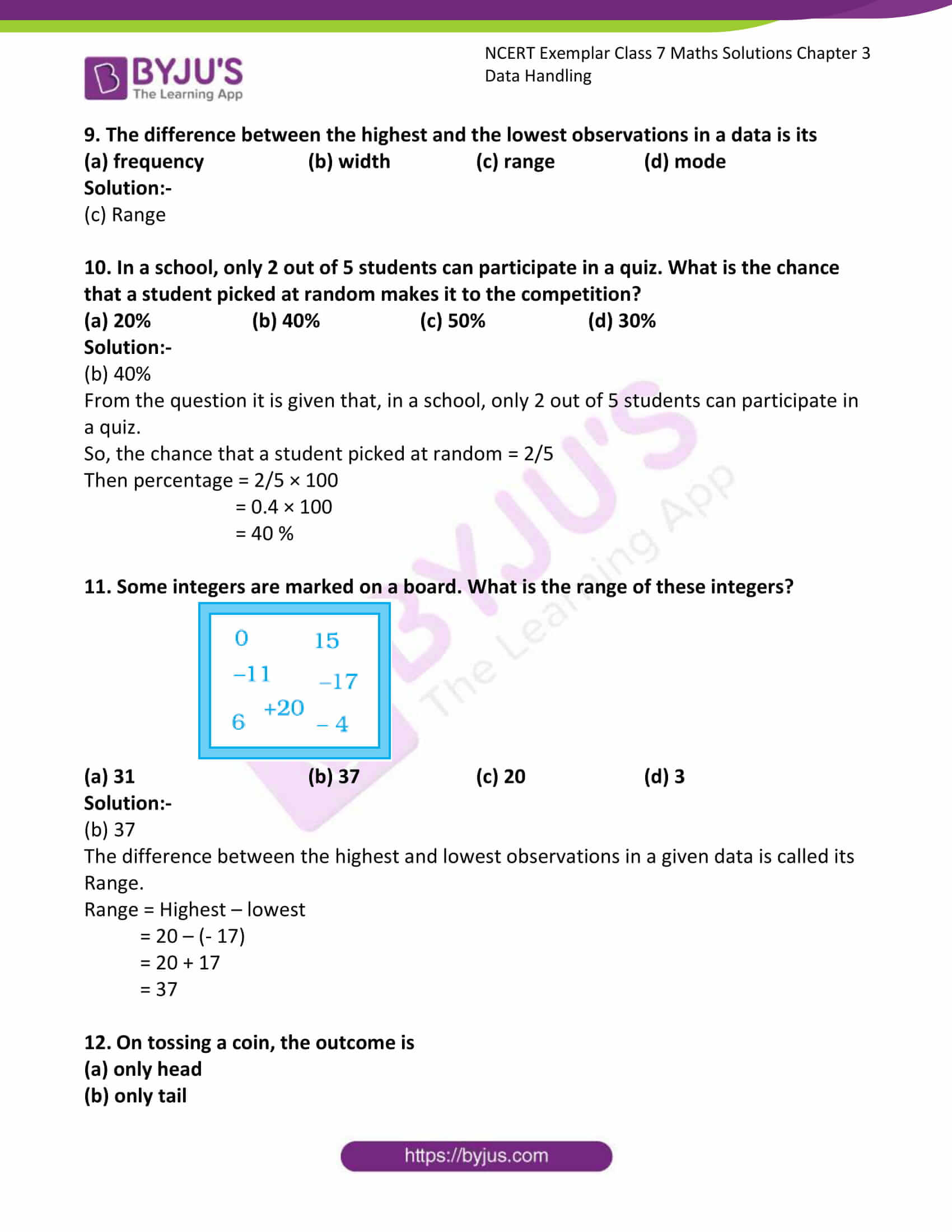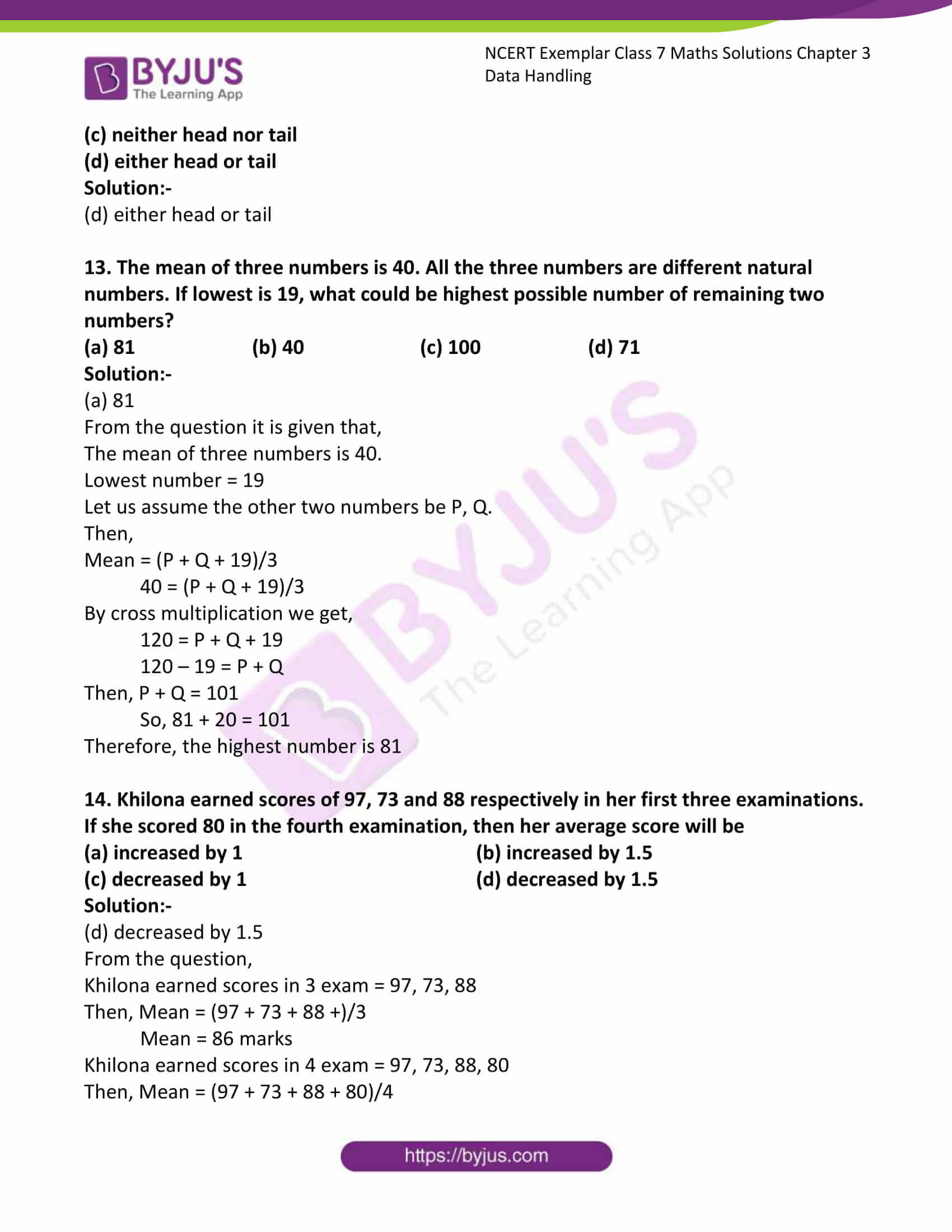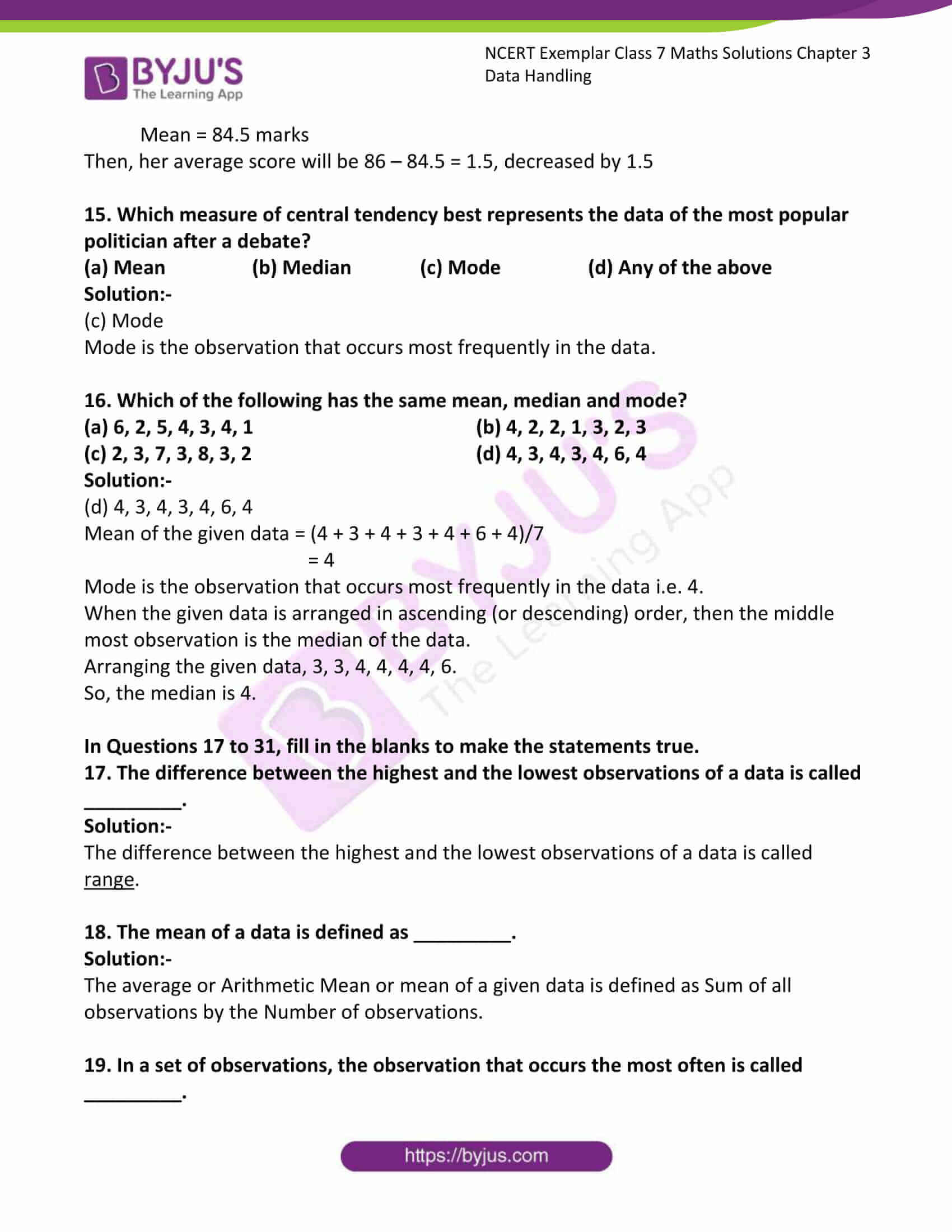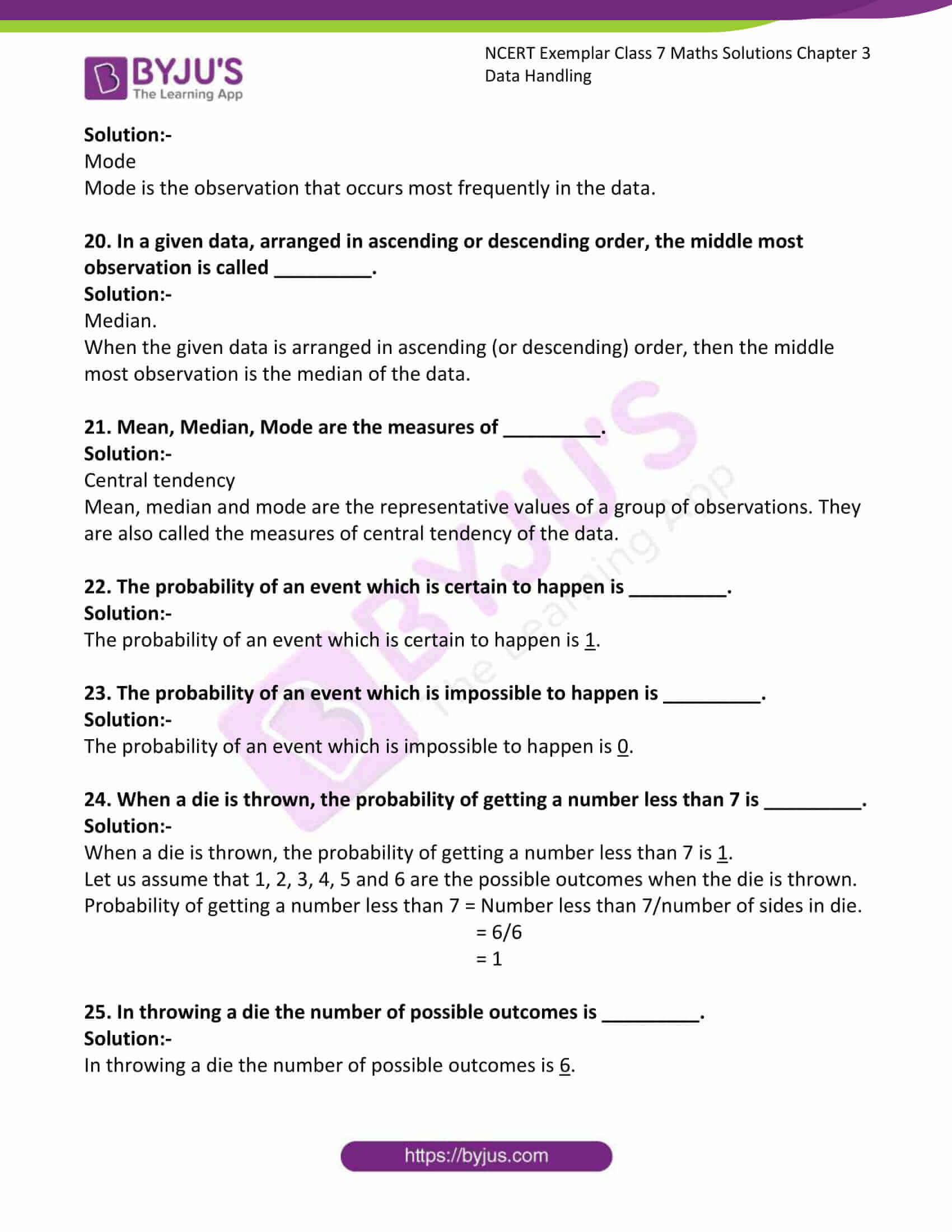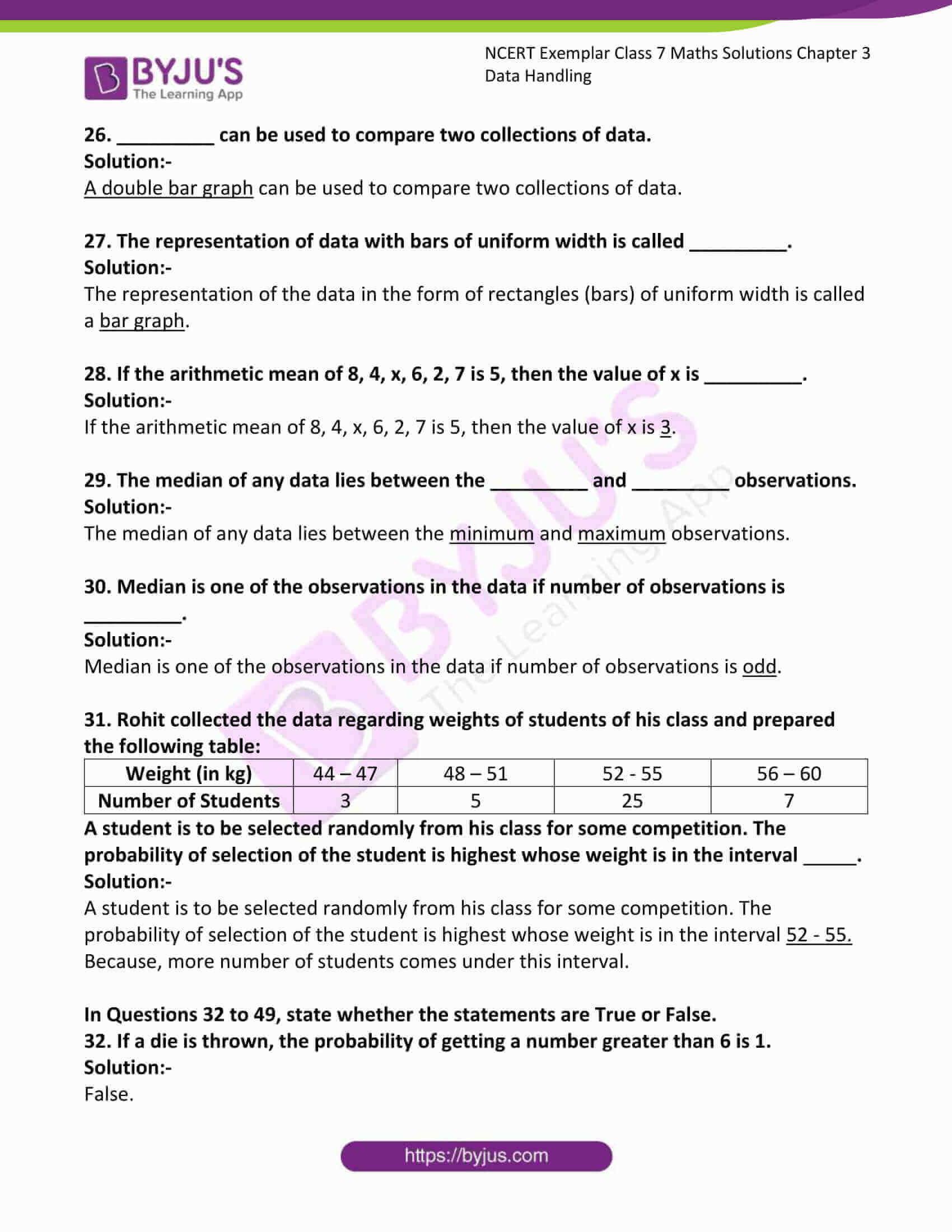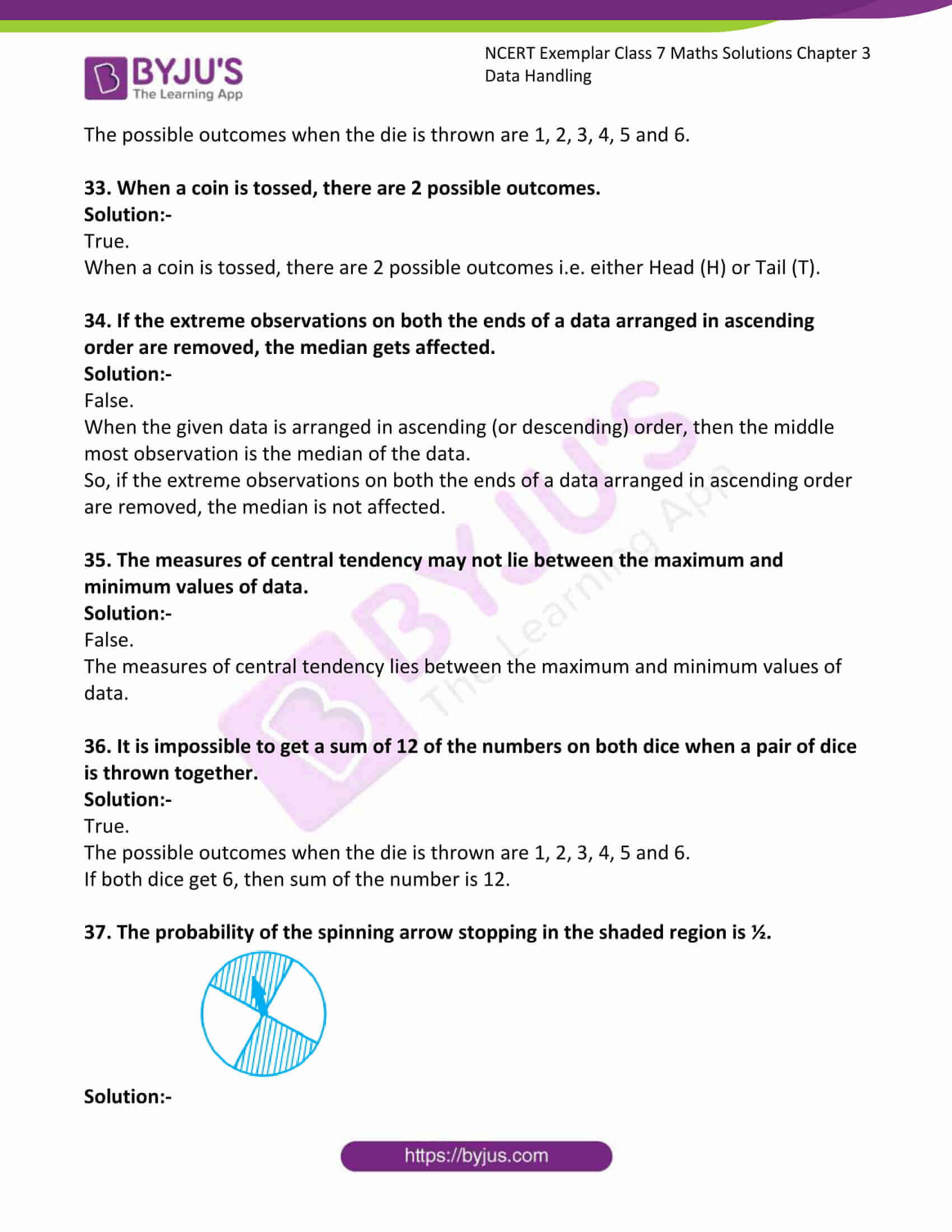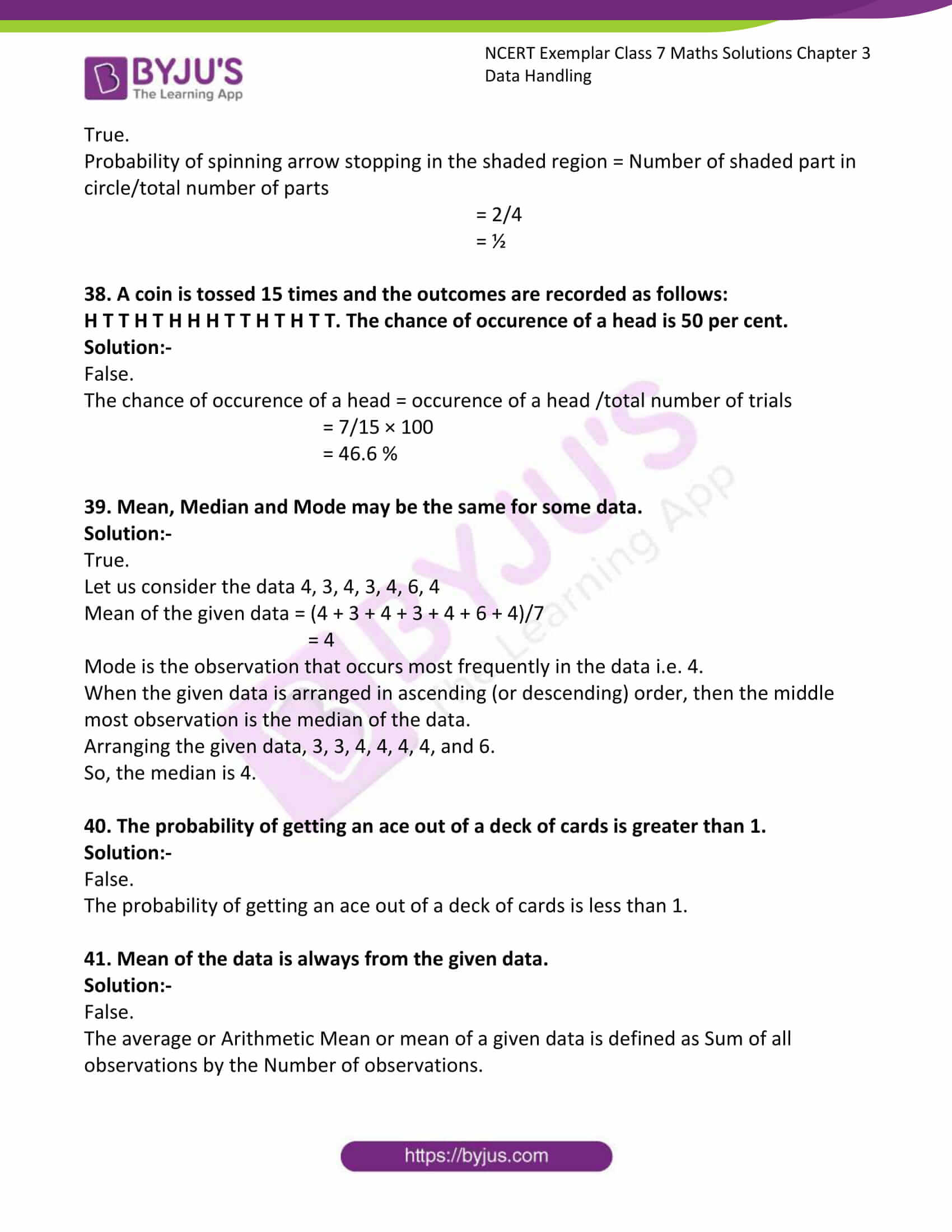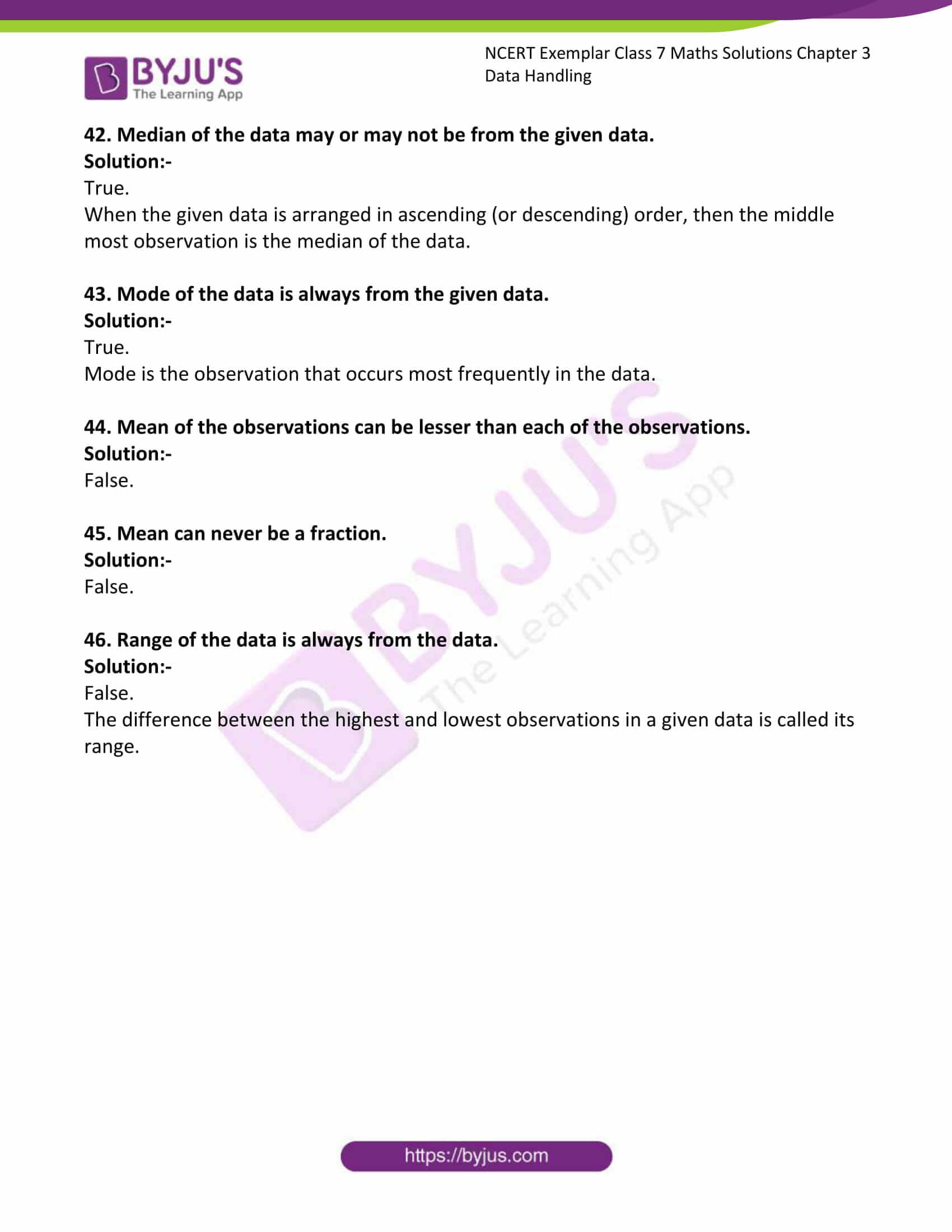### Access Answers to Maths NCERT Exemplar Solutions for Class 7 Chapter 3 Data Handling

Exercise Page: 72

In Questions 1 to 16, there are four options, out of which only one is correct. Write the correct answer.

1. Let x, y, z be three observations. The mean of these observations is

(a) (x × y × z)/3 (b) (x + y + z)/3
(c) (x – y – z)/3 (d) (x × y + z)/3

Solution:-

(b) (x + y + z)/3

The average or Arithmetic Mean or mean of a given data is defined as Sum of all observations by the Number of observations.

2. The number of trees in different parks of a city are 33, 38, 48, 33, 34, 34, 33 and 24. The mode of this data is

(a) 24 (b) 34 (c) 33 (d) 48

Solution:-

(c) 33

Mode is the observation that occurs most frequently in the data.

3. Which measures of central tendency get affected if the extreme observations on both the ends of a data arranged in descending order are removed?

(a) Mean and mode (b) Mean and Median

(c) Mode and Median (d) Mean, Median and Mode

Solution:-

(a) Mean and mode

4. The range of the data: 21, 6, 17, 18, 12, 8, 4, 13 is

(a) 17 (b) 12 (c) 8 (d) 15

Solution:-

(a) 17

The difference between the highest and lowest observations in a given data is called its Range.

Range = Highest – lowest

= 21 – 4

= 17

5. The median of the data: 3, 4, 5, 6, 7, 3, 4 is

(a) 5 (b) 3 (c) 4 (d) 6

Solution:-

(c) 4

When the given data is arranged in ascending (or descending) order, then the middle most observation is the median of the data.

So, now arrange the given observation into ascending order 3, 3, 4, 4, 5, 6, 7

Therefore, 4 is the middle most observation and it is the median of the data.

6. Out of 5 brands of chocolates in a shop, a boy has to purchase the brand which is most liked by children. What measure of central tendency would be most appropriate if the data is provided to him?

(a) Mean (b) Mode

(c) Median (d) Any of the three

Solution:-

(b) Mode

Mode is the observation that occurs most frequently in the data.

7. There are 2 aces in each of the given set of cards placed face down. From which set are you certain to pick the two aces in the first go?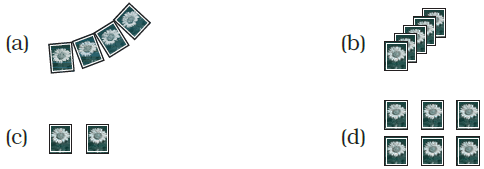Solution:-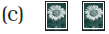The probability of picking the two aces in the first go is in option (c).

8. In the previous question, what is the probability of picking up an ace from set (d)?

(a) 1/6 (b) 2/6 (c) 3/6 (d) 4/6

Solution:-

(b) 2/6

Probability of picking up an ace from set = Number of ace in the set/ Total number of cards in the set.

9. The difference between the highest and the lowest observations in a data is its

(a) frequency (b) width (c) range (d) mode

Solution:-

(c) Range

10. In a school, only 2 out of 5 students can participate in a quiz. What is the chance that a student picked at random makes it to the competition?

(a) 20% (b) 40% (c) 50% (d) 30%

Solution:-

(b) 40%

From the question it is given that, in a school, only 2 out of 5 students can participate in a quiz.

So, the chance that a student picked at random = 2/5

Then percentage = 2/5 × 100

= 0.4 × 100

= 40 %

11. Some integers are marked on a board. What is the range of these integers?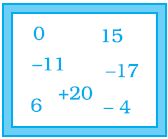(a) 31 (b) 37 (c) 20 (d) 3

Solution:-

(b) 37

The difference between the highest and lowest observations in a given data is called its Range.

Range = Highest – lowest

= 20 – (- 17)

= 20 + 17

= 37

12. On tossing a coin, the outcome is

(b) only tail

Solution:-

13. The mean of three numbers is 40. All the three numbers are different natural numbers. If lowest is 19, what could be highest possible number of remaining two numbers?

(a) 81 (b) 40 (c) 100 (d) 71

Solution:-

(a) 81

From the question it is given that,

The mean of three numbers is 40.

Lowest number = 19

Let us assume the other two numbers be P, Q.

Then,

Mean = (P + Q + 19)/3

40 = (P + Q + 19)/3

By cross multiplication we get,

120 = P + Q + 19

120 – 19 = P + Q

Then, P + Q = 101

So, 81 + 20 = 101

Therefore, the highest number is 81

14. Khilona earned scores of 97, 73 and 88 respectively in her first three examinations. If she scored 80 in the fourth examination, then her average score will be

(a) increased by 1 (b) increased by 1.5

(c) decreased by 1 (d) decreased by 1.5

Solution:-

(d) decreased by 1.5

From the question,

Khilona earned scores in 3 exam = 97, 73, 88

Then, Mean = (97 + 73 + 88 +)/3

Mean = 86 marks

Khilona earned scores in 4 exam = 97, 73, 88, 80

Then, Mean = (97 + 73 + 88 + 80)/4

Mean = 84.5 marks

Then, her average score will be 86 – 84.5 = 1.5, decreased by 1.5

15. Which measure of central tendency best represents the data of the most popular politician after a debate?

(a) Mean (b) Median (c) Mode (d) Any of the above

Solution:-

(c) Mode

Mode is the observation that occurs most frequently in the data.

16. Which of the following has the same mean, median and mode?

(a) 6, 2, 5, 4, 3, 4, 1 (b) 4, 2, 2, 1, 3, 2, 3

(c) 2, 3, 7, 3, 8, 3, 2 (d) 4, 3, 4, 3, 4, 6, 4

Solution:-

(d) 4, 3, 4, 3, 4, 6, 4

Mean of the given data = (4 + 3 + 4 + 3 + 4 + 6 + 4)/7

= 4

Mode is the observation that occurs most frequently in the data i.e. 4.

When the given data is arranged in ascending (or descending) order, then the middle most observation is the median of the data.

Arranging the given data, 3, 3, 4, 4, 4, 4, 6.

So, the median is 4.

In Questions 17 to 31, fill in the blanks to make the statements true.

17. The difference between the highest and the lowest observations of a data is called _________.

Solution:-

The difference between the highest and the lowest observations of a data is called range.

18. The mean of a data is defined as _________.

Solution:-

The average or Arithmetic Mean or mean of a given data is defined as Sum of all observations by the Number of observations.

19. In a set of observations, the observation that occurs the most often is called _________.

Solution:-

Mode

Mode is the observation that occurs most frequently in the data.

20. In a given data, arranged in ascending or descending order, the middle most observation is called _________.

Solution:-

Median.

When the given data is arranged in ascending (or descending) order, then the middle most observation is the median of the data.

21. Mean, Median, Mode are the measures of _________.

Solution:-

Central tendency

Mean, median and mode are the representative values of a group of observations. They are also called the measures of central tendency of the data.

22. The probability of an event which is certain to happen is _________.

Solution:-

The probability of an event which is certain to happen is 1.

23. The probability of an event which is impossible to happen is _________.

Solution:-

The probability of an event which is impossible to happen is 0.

24. When a die is thrown, the probability of getting a number less than 7 is _________.

Solution:-

When a die is thrown, the probability of getting a number less than 7 is 1.

Let us assume that 1, 2, 3, 4, 5 and 6 are the possible outcomes when the die is thrown.

Probability of getting a number less than 7 = Number less than 7/number of sides in die.

= 6/6

= 1

25. In throwing a die the number of possible outcomes is _________.

Solution:-

In throwing a die the number of possible outcomes is 6.

26. _________ can be used to compare two collections of data.

Solution:-

A double bar graph can be used to compare two collections of data.

27. The representation of data with bars of uniform width is called _________.

Solution:-

The representation of the data in the form of rectangles (bars) of uniform width is called a bar graph.

28. If the arithmetic mean of 8, 4, x, 6, 2, 7 is 5, then the value of x is _________.

Solution:-

If the arithmetic mean of 8, 4, x, 6, 2, 7 is 5, then the value of x is 3.

29. The median of any data lies between the _________ and _________ observations.

Solution:-

The median of any data lies between the minimum and maximum observations.

30. Median is one of the observations in the data if number of observations is _________.

Solution:-

Median is one of the observations in the data if number of observations is odd.

31. Rohit collected the data regarding weights of students of his class and prepared the following table:

 Weight (in kg) 44 – 47 48 – 51 52 – 55 56 – 60 Number of Students 3 5 25 7

A student is to be selected randomly from his class for some competition. The probability of selection of the student is highest whose weight is in the interval .

Solution:-

A student is to be selected randomly from his class for some competition. The probability of selection of the student is highest whose weight is in the interval 52 – 55.

Because, more number of students comes under this interval.

In Questions 32 to 49, state whether the statements are True or False.

32. If a die is thrown, the probability of getting a number greater than 6 is 1.

Solution:-

False.

The possible outcomes when the die is thrown are 1, 2, 3, 4, 5 and 6.

33. When a coin is tossed, there are 2 possible outcomes.

Solution:-

True.

When a coin is tossed, there are 2 possible outcomes i.e. either Head (H) or Tail (T).

34. If the extreme observations on both the ends of a data arranged in ascending order are removed, the median gets affected.

Solution:-

False.

When the given data is arranged in ascending (or descending) order, then the middle most observation is the median of the data.

So, if the extreme observations on both the ends of a data arranged in ascending order are removed, the median is not affected.

35. The measures of central tendency may not lie between the maximum and minimum values of data.

Solution:-

False.

The measures of central tendency lies between the maximum and minimum values of data.

36. It is impossible to get a sum of 12 of the numbers on both dice when a pair of dice is thrown together.

Solution:-

True.

The possible outcomes when the die is thrown are 1, 2, 3, 4, 5 and 6.

If both dice get 6, then sum of the number is 12.

37. The probability of the spinning arrow stopping in the shaded region is ½.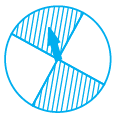Solution:-

True.

Probability of spinning arrow stopping in the shaded region = Number of shaded part in circle/total number of parts

= 2/4

= ½

38. A coin is tossed 15 times and the outcomes are recorded as follows:

H T T H T H H H T T H T H T T. The chance of occurence of a head is 50 per cent.

Solution:-

False.

The chance of occurence of a head = occurence of a head /total number of trials

= 7/15 × 100

= 46.6 %

39. Mean, Median and Mode may be the same for some data.

Solution:-

True.

Let us consider the data 4, 3, 4, 3, 4, 6, 4

Mean of the given data = (4 + 3 + 4 + 3 + 4 + 6 + 4)/7

= 4

Mode is the observation that occurs most frequently in the data i.e. 4.

When the given data is arranged in ascending (or descending) order, then the middle most observation is the median of the data.

Arranging the given data, 3, 3, 4, 4, 4, 4, and 6.

So, the median is 4.

40. The probability of getting an ace out of a deck of cards is greater than 1.

Solution:-

False.

The probability of getting an ace out of a deck of cards is less than 1.

41. Mean of the data is always from the given data.

Solution:-

False.

The average or Arithmetic Mean or mean of a given data is defined as Sum of all observations by the Number of observations.

42. Median of the data may or may not be from the given data.

Solution:-

True.

When the given data is arranged in ascending (or descending) order, then the middle most observation is the median of the data.

43. Mode of the data is always from the given data.

Solution:-

True.

Mode is the observation that occurs most frequently in the data.

44. Mean of the observations can be lesser than each of the observations.

Solution:-

False.

45. Mean can never be a fraction.

Solution:-

False.

46. Range of the data is always from the data.

Solution:-

False.

The difference between the highest and lowest observations in a given data is called its range.